﻿ N2MOH's Content - Netduino Forums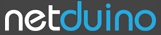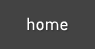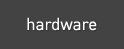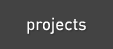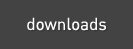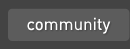The Netduino forums have been replaced by new forums at community.wildernesslabs.co. This site has been preserved for archival purposes only and the ability to make new accounts or posts has been turned off.

## N2MOH's Content

There have been 2 items by N2MOH (Search limited from 24-October 19)

#### See this member's

Sort by                Order

### #3354Simple Blinking LED Morse CodePosted by on 01 October 2010 - 01:21 PM in Project Showcase

Nice. IMHO using a lookup table would significantly increase readability and simplify the code - something like Scott Hanselman's sample (code is in the middle of the page, just above comments).

If I had seen Scott's post I wouldn't have bothered to post mine. Scott's code is smaller, thats true, but in my defence I will say that because of cut and paste I bet mine took less time to write.

Next time I will try to add something more interesting.

### #3272Simple Blinking LED Morse CodePosted by on 30 September 2010 - 12:50 PM in Project Showcase

Here is a short sample program that sends morse code by blinking the onboard LED
```using System;
using Microsoft.SPOT;
using Microsoft.SPOT.Hardware;
using SecretLabs.NETMF.Hardware;
using SecretLabs.NETMF.Hardware.Netduino;

namespace NetduinoApplication1 {
public class Program {
public static void Main() {
OutputPort led = new OutputPort(Pins.ONBOARD_LED, false);
sayhello sho = new sayhello();

while (true) {
sho.dosayhello(led);

}

}
}

public class sayhello {
private const int dittime = 100;
private const int dahtime = dittime*3;
private const int intersymtime = dittime * 1;
private const int interchartime = dittime * 5;
private const int interwordtime = dittime * 10;

public void dosayhello(OutputPort led) {
doWord(led, "HELLO");
doWord(led, "from");
doWord(led, "Greg");
}
public void doWord(OutputPort led, string ss) {
ss = ss.ToLower();

foreach (char cc in ss) {
switch (cc) {
case 'a': a(led); break;
case 'b': b(led); break;
case 'c': c(led); break;
case 'd': d(led); break;
case 'e': e(led); break;
case 'f': f(led); break;
case 'g': g(led); break;
case 'h': h(led); break;
case 'i': i(led); break;
case 'j': j(led); break;
case 'k': k(led); break;
case 'l': l(led); break;
case 'm': m(led); break;
case 'n': n(led); break;
case 'o': o(led); break;
case 'p': p(led); break;
case 'q': q(led); break;
case 'r': r(led); break;
case 's': s(led); break;
case 't': t(led); break;
case 'u': u(led); break;
case 'v': v(led); break;
case 'w': w(led); break;
case 'x': x(led); break;
case 'y': y(led); break;
case 'z': z(led); break;
}
}
}

private void a(OutputPort led) {
dit(led);
dah(led);
}
private void b(OutputPort led) {
dah(led);
dit(led);
dit(led);
dit(led);
}
private void c(OutputPort led) {
dah(led);
dit(led);
dah(led);
dit(led);
}
private void d(OutputPort led) {
dah(led);
dit(led);
dit(led);

}

private void e(OutputPort led) {
dit(led);
}
private void f(OutputPort led) {
dit(led); dit(led); dah(led); dit(led);
}
private void g(OutputPort led) {
dah(led); dah(led); dit(led);
}
private void h(OutputPort led) {
dit(led);
dit(led);
dit(led);
dit(led);
}
private void i(OutputPort led) {
dit(led);
dit(led);
}
private void j(OutputPort led) {
dit(led); dah(led); dah(led); dah(led);
}
private void k(OutputPort led) {
dah(led); dit(led); dah(led);
}
private void l(OutputPort led) {
dit(led);
dah(led);
dit(led);
dit(led);
}
private void m(OutputPort led) {
dah(led);
dah(led);
}
private void n(OutputPort led) {
dah(led);
dit(led);
}
private void o(OutputPort led) {
dah(led); dah(led); dah(led);
}
private void p(OutputPort led) {
dah(led); dah(led); dah(led); dah(led);
}
private void q(OutputPort led) {
dah(led); dah(led); dit(led); dah(led);
}
private void r(OutputPort led) {
dit(led); dah(led); dit(led);
}
private void s(OutputPort led) {
dit(led); dit(led); dit(led);
}
private void t(OutputPort led) {
}
private void u(OutputPort led) {
dit(led); dit(led); dah(led);
}
private void v(OutputPort led) {
dit(led); dit(led); dit(led); dah(led);
}
private void w(OutputPort led) {
dit(led); dah(led); dah(led);
}
private void x(OutputPort led) {
dah(led); dit(led); dit(led); dah(led);
}
private void y(OutputPort led) {
dah(led); dit(led); dah(led); dah(led);
}
private void z(OutputPort led) {
dah(led); dah(led); dit(led); dit(led);
}
private void dit(OutputPort led) {
led.Write(true);
led.Write(false);
}
private void dah(OutputPort led) {
led.Write(true);
led.Write(false);
}
}
}
```home    hardware    projects    downloads    community    where to buy    contact Copyright © 2016 Wilderness Labs Inc.  |  Legal   |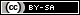This webpage is licensed under a Creative Commons Attribution-ShareAlike License.